LORENE
Lorene::Eos_compose_fit Class Reference

Equation of state for fitting the 1-parameter EoSs from the CompOSE database. More...

#include <eos_compose_fit.h>

Inheritance diagram for Lorene::Eos_compose_fit: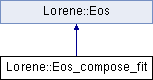## Public Member Functions

Eos_compose_fit (const string &param_file)
Constructor from a parameter file. More...

virtual ~Eos_compose_fit ()
Destructor. More...

virtual bool operator== (const Eos &) const
Comparison operator (egality) More...

virtual bool operator!= (const Eos &) const
Comparison operator (difference) More...

virtual int identify () const
Returns a number to identify the sub-classe of Eos the object belongs to. More...

virtual void sauve (FILE *) const
Save in a file. More...

void write_lorene_table (const string &, int nlines=200) const
Save into a table in Lorene format. More...

virtual double nbar_ent_p (double ent, const Param *par=0x0) const
Higher bound in density. More...

virtual double ener_ent_p (double ent, const Param *par=0x0) const
Computes the total energy density from the log-enthalpy. More...

virtual double press_ent_p (double ent, const Param *par=0x0) const
Computes the pressure from the log-enthalpy. More...

virtual double der_nbar_ent_p (double ent, const Param *par=0x0) const
Computes the logarithmic derivative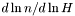from the log-enthalpy. More...

virtual double der_ener_ent_p (double ent, const Param *par=0x0) const
Computes the logarithmic derivative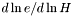from the log-enthalpy. More...

virtual double der_press_ent_p (double ent, const Param *par=0x0) const
Computes the logarithmic derivative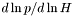from the log-enthalpy. More...

virtual double der_press_nbar_p (double ent, const Param *par=0x0) const
Computes the logarithmic derivative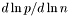from the log-enthalpy. More...

virtual double csound_square_ent_p (double, const Param *par=0x0) const
Computes the sound speed squared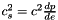from the enthapy with extra parameters (virtual function implemented in the derived classes). More...

const char * get_name () const
Returns the EOS name. More...

void set_name (const char *name_i)
Sets the EOS name. More...

Cmp nbar_ent (const Cmp &ent, int nzet, int l_min=0, Param *par=0x0) const
Computes the baryon density field from the log-enthalpy field and extra parameters. More...

Scalar nbar_ent (const Scalar &ent, int nzet, int l_min=0, Param *par=0x0) const
Computes the baryon density field from the log-enthalpy field and extra parameters. More...

Cmp ener_ent (const Cmp &ent, int nzet, int l_min=0, Param *par=0x0) const
Computes the total energy density from the log-enthalpy and extra parameters. More...

Scalar ener_ent (const Scalar &ent, int nzet, int l_min=0, Param *par=0x0) const
Computes the total energy density from the log-enthalpy and extra parameters. More...

Cmp press_ent (const Cmp &ent, int nzet, int l_min=0, Param *par=0x0) const
Computes the pressure from the log-enthalpy and extra parameters. More...

Scalar press_ent (const Scalar &ent, int nzet, int l_min=0, Param *par=0x0) const
Computes the pressure from the log-enthalpy and extra parameters. More...

Cmp der_nbar_ent (const Cmp &ent, int nzet, int l_min=0, Param *par=0x0) const
Computes the logarithmic derivativefrom the log-enthalpy and extra parameters. More...

Scalar der_nbar_ent (const Scalar &ent, int nzet, int l_min=0, Param *par=0x0) const
Computes the logarithmic derivativefrom the log-enthalpy and extra parameters. More...

Cmp der_ener_ent (const Cmp &ent, int nzet, int l_min=0, Param *par=0x0) const
Computes the logarithmic derivativefrom the log-enthalpy and extra parameters. More...

Scalar der_ener_ent (const Scalar &ent, int nzet, int l_min=0, Param *par=0x0) const
Computes the logarithmic derivativefrom the log-enthalpy and extra parameters. More...

Cmp der_press_ent (const Cmp &ent, int nzet, int l_min=0, Param *par=0x0) const
Computes the logarithmic derivativefrom the log-enthalpy and extra parameters. More...

Scalar der_press_ent (const Scalar &ent, int nzet, int l_min=0, Param *par=0x0) const
Computes the logarithmic derivativefrom the log-enthalpy and extra parameters. More...

Scalar csound_square_ent (const Scalar &ent, int nzet, int l_min=0, Param *par=0x0) const
Computes the sound speed squaredfrom the enthalpy with extra parameters. More...

## Static Public Member Functions

static Eoseos_from_file (FILE *)
Construction of an EOS from a binary file. More...

static Eoseos_from_file (ifstream &)
Construction of an EOS from a formatted file. More...

## Protected Member Functions

Eos_compose_fit (FILE *)
Constructor from a binary file (created by the function sauve(FILE*) ). More...

Eos_compose_fit (ifstream &)
Constructor from a formatted file. More...

Reads the Compose data and makes the fit. More...

void read_compose_data (int &nbp, Tbl *&logh, Tbl *&logp, Tbl *&loge, Tbl *&lognb, Tbl *&gam1)
Reads Compose data and stores the values of thermodynamic quantities. More...

From the read values, makes the fit on the adiabatic index and deduces the other quantities from thermodynamic relations and definitions. More...

double integrate_equations (double kappa, double mean_gam, const Scalar &gamma1, const Scalar &log_ent, const Scalar &ent, Scalar &YYY)
Integrates the differential system giving all thermo quantities from the adiabatic index. More...

virtual ostream & operator>> (ostream &) const
Operator >> More...

const string & get_tablename () const
Returns the name (given in the parameter file, see the introduction of the class). More...

double get_nbmin () const

double get_nbmax () const
Lower bound in density. More...

void calcule (const Cmp &thermo, int nzet, int l_min, double(Eos::*fait)(double, const Param *) const, Param *par, Cmp &resu) const
General computational method for Cmp 's. More...

void calcule (const Scalar &thermo, int nzet, int l_min, double(Eos::*fait)(double, const Param *) const, Param *par, Scalar &resu) const
General computational method for Scalar 's. More...

## Protected Attributes

string tablename
Name of the file containing the tabulated data. More...

int n_coefs
Number of coeficients for polynomial regression. More...

double nb_min
Lower bound in baryon density, below which the EoS is assumed to be a polytrope. More...

double nb_mid
Middle bound in baryon density, above which which the EoS is determined from the polynomial fit to the adiabatic index. More...

double nb_max
Higher bound on the density, above which the EoS is assumed to be a polytrope. More...

double hmin
Values of enthalpy corresponding to nb_min and nb_max. More...

double hmax

const Eos_polyp_eos_low
Pointer on a polytropic EoS for the description of low densities (nb<nb_min) More...

const Eos_polyp_eos_high
Pointer on a polytropic EoS for the description of high densities (nb>nb_max) More...

const Mg3dmg
Multi-grid defining the number of domains and spectral coefficients. More...

const Map_afmp
Mapping in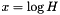. More...

Scalarlog_p
Table of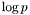. More...

Scalarlog_e
Table of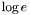. More...

Scalarlog_nb
Table of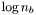. More...

Scalarlog_cs2
Table of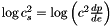. More...

char name 
EOS name. More...

## Private Member Functions

Eos_compose_fit (const Eos_compose_fit &)
Copy constructor (private to make Eos_compose_fit a non-copiable class) More...

## Friends

EosEos::eos_from_file (FILE *)
The construction functions from a file. More...

EosEos::eos_from_file (ifstream &)

## Detailed Description

Equation of state for fitting the 1-parameter EoSs from the CompOSE database.

()

A polynomial fit is done ont the adidabatic index for the highest densities. At the lowest ones, a simple polytrope is fitted. In between, a linear interpolation is done in terms of adiabatic index. When built with Eos::eos_from_file(), the file must be composed of the following lines:

7 Type of the EOS
EoS fitted from CompOSE data
/full/path/to/the/eos/table/
0.01   1.e-9  # limiting density values
6             # polynomial degree for the regression
33            # number of Chebyshev point sin each domain 

On the second line the name is just for output. The path to the directory containing CompOSE EoS (third line), does not contain the name of the tables, it is assumed to be called eos.nb and eos.thermo (see CompOSE documentation). The fourth line gives limit values in density for the fit: above the first value, the polynomial regression is done. Below the second value, a polytrope is fitted.

Definition at line 90 of file eos_compose_fit.h.

## ◆ Eos_compose_fit() [1/4]

 Lorene::Eos_compose_fit::Eos_compose_fit ( const string & param_file )

Constructor from a parameter file.

Parameters
 files_path Absolute name (including path) of the parameter file.

Definition at line 71 of file eos_compose_fit.C.

## ◆ Eos_compose_fit() [2/4]

 Lorene::Eos_compose_fit::Eos_compose_fit ( FILE * fich )
protected

Constructor from a binary file (created by the function sauve(FILE*) ).

This constructor is protected because any EOS construction from a binary file must be done via the function Eos::eos_from_file(FILE*) .

Definition at line 86 of file eos_compose_fit.C.

References Lorene::fread_be(), hmin, log_cs2, log_e, log_nb, log_p, mg, mp, n_coefs, nb_max, nb_mid, nb_min, p_eos_high, p_eos_low, and tablename.

## ◆ Eos_compose_fit() [3/4]

 Lorene::Eos_compose_fit::Eos_compose_fit ( ifstream & para_file )
protected

Constructor from a formatted file.

This constructor is protected because any EOS construction from a formatted file must be done via the function Eos::eos_from_file(ifstream& ) .

Definition at line 129 of file eos_compose_fit.C.

## ◆ Eos_compose_fit() [4/4]

 Lorene::Eos_compose_fit::Eos_compose_fit ( const Eos_compose_fit & )
private

Copy constructor (private to make Eos_compose_fit a non-copiable class)

## ◆ ~Eos_compose_fit()

 Lorene::Eos_compose_fit::~Eos_compose_fit ( )
virtual

Destructor.

Definition at line 142 of file eos_compose_fit.C.

References log_cs2, log_e, log_nb, log_p, mg, mp, p_eos_high, and p_eos_low.

## Member Function Documentation

protected

From the read values, makes the fit on the adiabatic index and deduces the other quantities from thermodynamic relations and definitions.

Definition at line 200 of file eos_compose_fit_build.C.

## ◆ calcule() [1/2]

 void Lorene::Eos::calcule ( const Cmp & thermo, int nzet, int l_min, double(Eos::*)(double, const Param *) const fait, Param * par, Cmp & resu ) const
protectedinherited

General computational method for Cmp 's.

Parameters
 thermo [input] thermodynamical quantity (for instance the enthalpy field)from which the thermodynamical quantity resu is to be computed. nzet [input] number of domains where resu is to be computed. l_min [input] index of the innermost domain is which resu is to be computed [default value: 0]; resu is computed only in domains whose indices are in [l_min,l_min+nzet-1] . In the other domains, it is set to zero. fait [input] pointer on the member function of class Eos which performs the pointwise calculation. par possible extra parameters of the EOS resu [output] result of the computation.

Definition at line 213 of file eos.C.

References Lorene::Cmp::get_etat().

## ◆ calcule() [2/2]

 void Lorene::Eos::calcule ( const Scalar & thermo, int nzet, int l_min, double(Eos::*)(double, const Param *) const fait, Param * par, Scalar & resu ) const
protectedinherited

General computational method for Scalar 's.

Parameters
 thermo [input] thermodynamical quantity (for instance the enthalpy field)from which the thermodynamical quantity resu is to be computed. nzet [input] number of domains where resu is to be computed. l_min [input] index of the innermost domain is which resu is to be computed [default value: 0]; resu is computed only in domains whose indices are in [l_min,l_min+nzet-1] . In the other domains, it is set to zero. fait [input] pointer on the member function of class Eos which performs the pointwise calculation. par possible extra parameters of the EOS resu [output] result of the computation.

Definition at line 285 of file eos.C.

References Lorene::Scalar::get_etat().

## ◆ csound_square_ent()

 Scalar Lorene::Eos::csound_square_ent ( const Scalar & ent, int nzet, int l_min = 0, Param * par = 0x0 ) const
inherited

Computes the sound speed squaredfrom the enthalpy with extra parameters.

Parameters
 ent [input, unit: c^2] enthalpy nzet number of domains where the derivative dln(e)/dln(H) is to be computed. l_min index of the innermost domain is which the coefficient dln(n)/dln(H) is to be computed [default value: 0]; the derivative dln(e)/dln(H) is computed only in domains whose indices are in [l_min,l_min+nzet-1] . In the other domains, it is set to zero. par possible extra parameters of the EOS
Returns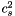[unit: c^2]

Definition at line 499 of file eos.C.

## ◆ csound_square_ent_p()

 double Lorene::Eos_compose_fit::csound_square_ent_p ( double ent, const Param * par = 0x0 ) const
virtual

Computes the sound speed squaredfrom the enthapy with extra parameters (virtual function implemented in the derived classes).

Parameters
 ent [input, unit: c^2] enthalpy par possible extra parameters of the EOS
Returns[unit: c^2]

Implements Lorene::Eos.

Definition at line 481 of file eos_compose_fit.C.

## ◆ der_ener_ent() [1/2]

 Cmp Lorene::Eos::der_ener_ent ( const Cmp & ent, int nzet, int l_min = 0, Param * par = 0x0 ) const
inherited

Computes the logarithmic derivativefrom the log-enthalpy and extra parameters.

Parameters
 ent [input, unit: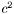] log-enthalpy H defined by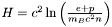, where e is the (total) energy density, p the pressure, n the baryon density, and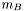the baryon mass nzet number of domains where the derivative dln(e)/dln(H) is to be computed. l_min index of the innermost domain is which the coefficient dln(n)/dln(H) is to be computed [default value: 0]; the derivative dln(e)/dln(H) is computed only in domains whose indices are in [l_min,l_min+nzet-1] . In the other domains, it is set to zero. par possible extra parameters of the EOS
Returns
dln(e)/dln(H)

Definition at line 454 of file eos.C.

## ◆ der_ener_ent() [2/2]

 Scalar Lorene::Eos::der_ener_ent ( const Scalar & ent, int nzet, int l_min = 0, Param * par = 0x0 ) const
inherited

Computes the logarithmic derivativefrom the log-enthalpy and extra parameters.

Parameters
 ent [input, unit:] log-enthalpy H defined by, where e is the (total) energy density, p the pressure, n the baryon density, andthe baryon mass nzet number of domains where the derivative dln(e)/dln(H) is to be computed. l_min index of the innermost domain is which the coefficient dln(n)/dln(H) is to be computed [default value: 0]; the derivative dln(e)/dln(H) is computed only in domains whose indices are in [l_min,l_min+nzet-1] . In the other domains, it is set to zero. par possible extra parameters of the EOS
Returns
dln(e)/dln(H)

Definition at line 464 of file eos.C.

## ◆ der_ener_ent_p()

 double Lorene::Eos_compose_fit::der_ener_ent_p ( double ent, const Param * par = 0x0 ) const
virtual

Computes the logarithmic derivativefrom the log-enthalpy.

Parameters
 ent [input, unit:] log-enthalpy H
Returns
dln(e)/dln(H)

Implements Lorene::Eos.

Definition at line 446 of file eos_compose_fit.C.

References csound_square_ent_p(), ener_ent_p(), and press_ent_p().

## ◆ der_nbar_ent() [1/2]

 Cmp Lorene::Eos::der_nbar_ent ( const Cmp & ent, int nzet, int l_min = 0, Param * par = 0x0 ) const
inherited

Computes the logarithmic derivativefrom the log-enthalpy and extra parameters.

Parameters
 ent [input, unit:] log-enthalpy H defined by, where e is the (total) energy density, p the pressure, n the baryon density, andthe baryon mass nzet number of domains where the derivative dln(n)/dln(H) is to be computed. l_min index of the innermost domain is which the coefficient dln(n)/dln(H) is to be computed [default value: 0]; the derivative dln(n)/dln(H) is computed only in domains whose indices are in [l_min,l_min+nzet-1] . In the other domains, it is set to zero. par possible extra parameters of the EOS
Returns
dln(n)/dln(H)

Definition at line 431 of file eos.C.

## ◆ der_nbar_ent() [2/2]

 Scalar Lorene::Eos::der_nbar_ent ( const Scalar & ent, int nzet, int l_min = 0, Param * par = 0x0 ) const
inherited

Computes the logarithmic derivativefrom the log-enthalpy and extra parameters.

Parameters
 ent [input, unit:] log-enthalpy H defined by, where e is the (total) energy density, p the pressure, n the baryon density, andthe baryon mass nzet number of domains where the derivative dln(n)/dln(H) is to be computed. l_min index of the innermost domain is which the coefficient dln(n)/dln(H) is to be computed [default value: 0]; the derivative dln(n)/dln(H) is computed only in domains whose indices are in [l_min,l_min+nzet-1] . In the other domains, it is set to zero. par possible extra parameters of the EOS
Returns
dln(n)/dln(H)

Definition at line 441 of file eos.C.

## ◆ der_nbar_ent_p()

 double Lorene::Eos_compose_fit::der_nbar_ent_p ( double ent, const Param * par = 0x0 ) const
virtual

Computes the logarithmic derivativefrom the log-enthalpy.

Parameters
 ent [input, unit:] log-enthalpy H
Returns
dln(n)/dln(H)

Implements Lorene::Eos.

Definition at line 437 of file eos_compose_fit.C.

References csound_square_ent_p().

## ◆ der_press_ent() [1/2]

 Cmp Lorene::Eos::der_press_ent ( const Cmp & ent, int nzet, int l_min = 0, Param * par = 0x0 ) const
inherited

Computes the logarithmic derivativefrom the log-enthalpy and extra parameters.

Parameters
 ent [input, unit:] log-enthalpy H defined by, where e is the (total) energy density, p the pressure, n the baryon density, andthe baryon mass nzet number of domains where the derivative dln(p)/dln(H) is to be computed. par possible extra parameters of the EOS l_min index of the innermost domain is which the coefficient dln(n)/dln(H) is to be computed [default value: 0]; the derivative dln(p)/dln(H) is computed only in domains whose indices are in [l_min,l_min+nzet-1] . In the other domains, it is set to zero.
Returns
dln(p)/dln(H)

Definition at line 476 of file eos.C.

## ◆ der_press_ent() [2/2]

 Scalar Lorene::Eos::der_press_ent ( const Scalar & ent, int nzet, int l_min = 0, Param * par = 0x0 ) const
inherited

Computes the logarithmic derivativefrom the log-enthalpy and extra parameters.

Parameters
 ent [input, unit:] log-enthalpy H defined by, where e is the (total) energy density, p the pressure, n the baryon density, andthe baryon mass nzet number of domains where the derivative dln(p)/dln(H) is to be computed. par possible extra parameters of the EOS l_min index of the innermost domain is which the coefficient dln(n)/dln(H) is to be computed [default value: 0]; the derivative dln(p)/dln(H) is computed only in domains whose indices are in [l_min,l_min+nzet-1] . In the other domains, it is set to zero.
Returns
dln(p)/dln(H)

Definition at line 486 of file eos.C.

## ◆ der_press_ent_p()

 double Lorene::Eos_compose_fit::der_press_ent_p ( double ent, const Param * par = 0x0 ) const
virtual

Computes the logarithmic derivativefrom the log-enthalpy.

Parameters
 ent [input, unit:] log-enthalpy H
Returns
dln(p)/dln(H)

Implements Lorene::Eos.

Definition at line 459 of file eos_compose_fit.C.

References ener_ent_p(), and press_ent_p().

## ◆ der_press_nbar_p()

 double Lorene::Eos_compose_fit::der_press_nbar_p ( double ent, const Param * par = 0x0 ) const
virtual

Computes the logarithmic derivativefrom the log-enthalpy.

Parameters
 ent [input, unit:] log-enthalpy H
Returns
dln(p)/dln(n)

Definition at line 472 of file eos_compose_fit.C.

References der_nbar_ent_p(), and der_press_ent_p().

## ◆ ener_ent() [1/2]

 Cmp Lorene::Eos::ener_ent ( const Cmp & ent, int nzet, int l_min = 0, Param * par = 0x0 ) const
inherited

Computes the total energy density from the log-enthalpy and extra parameters.

Parameters
 ent [input, unit:] log-enthalpy H defined by, where e is the (total) energy density, p the pressure, n the baryon density, andthe baryon mass nzet number of domains where the energy density is to be computed. l_min index of the innermost domain is which the energy density is to be computed [default value: 0]; the energy density is computed only in domains whose indices are in [l_min,l_min+nzet-1] . In the other domains, it is set to zero. par possible extra parameters of the EOS
Returns
energy density [unit: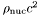], where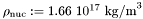Definition at line 387 of file eos.C.

References Lorene::Eos::calcule(), Lorene::Eos::ener_ent_p(), and Lorene::Cmp::get_mp().

## ◆ ener_ent() [2/2]

 Scalar Lorene::Eos::ener_ent ( const Scalar & ent, int nzet, int l_min = 0, Param * par = 0x0 ) const
inherited

Computes the total energy density from the log-enthalpy and extra parameters.

Parameters
 ent [input, unit:] log-enthalpy H defined by, where e is the (total) energy density, p the pressure, n the baryon density, andthe baryon mass nzet number of domains where the energy density is to be computed. l_min index of the innermost domain is which the energy density is to be computed [default value: 0]; the energy density is computed only in domains whose indices are in [l_min,l_min+nzet-1] . In the other domains, it is set to zero. par possible extra parameters of the EOS
Returns
energy density [unit:], whereDefinition at line 397 of file eos.C.

## ◆ ener_ent_p()

 double Lorene::Eos_compose_fit::ener_ent_p ( double ent, const Param * par = 0x0 ) const
virtual

Computes the total energy density from the log-enthalpy.

Parameters
 ent [input, unit:] log-enthalpy H
Returns
energy density e [unit:], whereImplements Lorene::Eos.

Definition at line 395 of file eos_compose_fit.C.

## ◆ eos_from_file() [1/2]

 Eos * Lorene::Eos::eos_from_file ( FILE * fich )
staticinherited

Construction of an EOS from a binary file.

The file must have been created by the function sauve(FILE*) .

Definition at line 193 of file eos_from_file.C.

## ◆ eos_from_file() [2/2]

 Eos * Lorene::Eos::eos_from_file ( ifstream & fich )
staticinherited

Construction of an EOS from a formatted file.

The fist line of the file must start by the EOS number, according to the following conventions:

• 1 = relativistic polytropic EOS (class Eos_poly ).
• 2 = Newtonian polytropic EOS (class Eos_poly_newt ).
• 3 = Relativistic incompressible EOS (class Eos_incomp ).
• 4 = Newtonian incompressible EOS (class Eos_incomp_newt ).
• 5 = Strange matter (MIT Bag model)
• 6 = Strange matter (MIT Bag model) with crust
• 10 = SLy4 (Douchin & Haensel 2001)
- 11 = FPS (Friedman-Pandharipande + Skyrme)
• 12 = BPAL12 (Bombaci et al. 1995)
• 13 = AkmalPR (Akmal, Pandharipande & Ravenhall 1998)
• 14 = BBB2 (Baldo, Bombaci & Burgio 1997)
• 15 = BalbN1H1 (Balberg 2000)
• 16 = GlendNH3 (Glendenning 1985, case 3)
• 17 = Tabulated EOS in CompOSE format
• 18 = magnetized (tabulated) equation of state
• 19 = relativistic ideal Fermi gas at zero temperature (class Eos_Fermi)
• 20 = Tabulated EOS in CompOSE format corrected for thermo. consistency
• 100 = Multi-domain EOS (class MEos )
• 110 = Multi-polytropic EOS (class Eos_multi_poly )
• 120 = Fitted SLy4 (Shibata 2004)
• 121 = Fitted FPS (Shibata 2004)
• 122 = Fitted AkmalPR (Taniguchi 2005)

The second line in the file should contain a name given by the user to the EOS. The following lines should contain the EOS parameters (one parameter per line), in the same order than in the class declaration.

Definition at line 345 of file eos_from_file.C.

## ◆ get_name()

 const char * Lorene::Eos::get_name ( ) const
inherited

Returns the EOS name.

Definition at line 179 of file eos.C.

References Lorene::Eos::name.

## ◆ get_nbmax()

 double Lorene::Eos_compose_fit::get_nbmax ( ) const
inlineprotected

Lower bound in density.

Definition at line 243 of file eos_compose_fit.h.

References nb_max.

## ◆ get_tablename()

 const string& Lorene::Eos_compose_fit::get_tablename ( ) const
inlineprotected

Returns the name (given in the parameter file, see the introduction of the class).

Definition at line 240 of file eos_compose_fit.h.

References tablename.

## ◆ identify()

 int Lorene::Eos_compose_fit::identify ( ) const
virtual

Returns a number to identify the sub-classe of Eos the object belongs to.

Implements Lorene::Eos.

Definition at line 151 of file eos_from_file.C.

## ◆ integrate_equations()

 double Lorene::Eos_compose_fit::integrate_equations ( double kappa, double mean_gam, const Scalar & gamma1, const Scalar & log_ent, const Scalar & ent, Scalar & YYY )
protected

Integrates the differential system giving all thermo quantities from the adiabatic index.

Returns
pressure at the highest entropy of the original table

Definition at line 364 of file eos_compose_fit_build.C.

## ◆ nbar_ent() [1/2]

 Cmp Lorene::Eos::nbar_ent ( const Cmp & ent, int nzet, int l_min = 0, Param * par = 0x0 ) const
inherited

Computes the baryon density field from the log-enthalpy field and extra parameters.

Parameters
 ent [input, unit:] log-enthalpy H defined by, where e is the (total) energy density, p the pressure, n the baryon density, andthe baryon mass nzet number of domains where the baryon density is to be computed. l_min index of the innermost domain is which the baryon density is to be computed [default value: 0]; the baryon density is computed only in domains whose indices are in [l_min,l_min+nzet-1] . In the other domains, it is set to zero. par possible extra parameters of the EOS
Returns
baryon density [unit: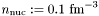]

Definition at line 362 of file eos.C.

References Lorene::Eos::calcule(), Lorene::Cmp::get_mp(), and Lorene::Eos::nbar_ent_p().

## ◆ nbar_ent() [2/2]

 Scalar Lorene::Eos::nbar_ent ( const Scalar & ent, int nzet, int l_min = 0, Param * par = 0x0 ) const
inherited

Computes the baryon density field from the log-enthalpy field and extra parameters.

Parameters
 ent [input, unit:] log-enthalpy H defined by, where e is the (total) energy density, p the pressure, n the baryon density, andthe baryon mass nzet number of domains where the baryon density is to be computed. l_min index of the innermost domain is which the baryon density is to be computed [default value: 0]; the baryon density is computed only in domains whose indices are in [l_min,l_min+nzet-1] . In the other domains, it is set to zero. par possible extra parameters of the EOS
Returns
baryon density [unit:]

Definition at line 372 of file eos.C.

## ◆ nbar_ent_p()

 double Lorene::Eos_compose_fit::nbar_ent_p ( double ent, const Param * par = 0x0 ) const
virtual

Higher bound in density.

Computes the baryon density from the log-enthalpy.

Parameters
 ent [input, unit:] log-enthalpy H
Returns
baryon density n [unit:]

Implements Lorene::Eos.

Definition at line 374 of file eos_compose_fit.C.

## ◆ operator!=()

 bool Lorene::Eos_compose_fit::operator!= ( const Eos & eos_i ) const
virtual

Comparison operator (difference)

Implements Lorene::Eos.

Definition at line 169 of file eos_compose_fit.C.

References operator==().

## ◆ operator==()

 bool Lorene::Eos_compose_fit::operator== ( const Eos & eos_i ) const
virtual

Comparison operator (egality)

Implements Lorene::Eos.

Definition at line 158 of file eos_compose_fit.C.

References identify(), and Lorene::Eos::identify().

## ◆ operator>>()

 ostream & Lorene::Eos_compose_fit::operator>> ( ostream & ost ) const
protectedvirtual

Operator >>

Implements Lorene::Eos.

Definition at line 218 of file eos_compose_fit.C.

References Lorene::exp(), hmin, mg, mp, n_coefs, nb_max, nb_mid, nb_min, p_eos_high, p_eos_low, tablename, and Lorene::Map_af::val_r_jk().

## ◆ press_ent() [1/2]

 Cmp Lorene::Eos::press_ent ( const Cmp & ent, int nzet, int l_min = 0, Param * par = 0x0 ) const
inherited

Computes the pressure from the log-enthalpy and extra parameters.

Parameters
 ent [input, unit:] log-enthalpy H defined by, where e is the (total) energy density, p the pressure, n the baryon density, andthe baryon mass nzet number of domains where the pressure is to be computed. l_min index of the innermost domain is which the pressure is to be computed [default value: 0]; the pressure is computed only in domains whose indices are in [l_min,l_min+nzet-1] . In the other domains, it is set to zero. par possible extra parameters of the EOS
Returns
pressure [unit:], whereDefinition at line 409 of file eos.C.

## ◆ press_ent() [2/2]

 Scalar Lorene::Eos::press_ent ( const Scalar & ent, int nzet, int l_min = 0, Param * par = 0x0 ) const
inherited

Computes the pressure from the log-enthalpy and extra parameters.

Parameters
 ent [input, unit:] log-enthalpy H defined by, where e is the (total) energy density, p the pressure, n the baryon density, andthe baryon mass nzet number of domains where the pressure is to be computed. l_min index of the innermost domain is which the pressure is to be computed [default value: 0]; the pressure is computed only in domains whose indices are in [l_min,l_min+nzet-1] . In the other domains, it is set to zero. par possible extra parameters of the EOS
Returns
pressure [unit:], whereDefinition at line 419 of file eos.C.

## ◆ press_ent_p()

 double Lorene::Eos_compose_fit::press_ent_p ( double ent, const Param * par = 0x0 ) const
virtual

Computes the pressure from the log-enthalpy.

Parameters
 ent [input, unit:] log-enthalpy H
Returns
pressure p [unit:], whereImplements Lorene::Eos.

Definition at line 416 of file eos_compose_fit.C.

 void Lorene::Eos_compose_fit::read_and_compute ( ifstream & para_file )
protected

Reads the Compose data and makes the fit.

Definition at line 239 of file eos_compose_fit.C.

 void Lorene::Eos_compose_fit::read_compose_data ( int & nbp, Tbl *& logh, Tbl *& logp, Tbl *& loge, Tbl *& lognb, Tbl *& gam1 )
protected

Reads Compose data and stores the values of thermodynamic quantities.

Definition at line 63 of file eos_compose_fit_build.C.

## ◆ sauve()

 void Lorene::Eos_compose_fit::sauve ( FILE * fich ) const
virtual

Save in a file.

Reimplemented from Lorene::Eos.

Definition at line 178 of file eos_compose_fit.C.

## ◆ set_name()

 void Lorene::Eos::set_name ( const char * name_i )
inherited

Sets the EOS name.

Definition at line 173 of file eos.C.

References Lorene::Eos::name.

## ◆ write_lorene_table()

 void Lorene::Eos_compose_fit::write_lorene_table ( const string & name_file, int nlines = 200 ) const

Save into a table in Lorene format.

Definition at line 301 of file eos_compose_fit.C.

## ◆ Eos::eos_from_file

 Eos* Eos::eos_from_file ( FILE * )
friend

The construction functions from a file.

## ◆ hmin

 double Lorene::Eos_compose_fit::hmin
protected

Values of enthalpy corresponding to nb_min and nb_max.

Definition at line 117 of file eos_compose_fit.h.

## ◆ log_cs2

 Scalar* Lorene::Eos_compose_fit::log_cs2
protected

Table of.

Definition at line 141 of file eos_compose_fit.h.

## ◆ log_e

 Scalar* Lorene::Eos_compose_fit::log_e
protected

Table of.

Definition at line 135 of file eos_compose_fit.h.

## ◆ log_nb

 Scalar* Lorene::Eos_compose_fit::log_nb
protected

Table of.

Definition at line 138 of file eos_compose_fit.h.

## ◆ log_p

 Scalar* Lorene::Eos_compose_fit::log_p
protected

Table of.

Definition at line 132 of file eos_compose_fit.h.

## ◆ mg

 const Mg3d* Lorene::Eos_compose_fit::mg
protected

Multi-grid defining the number of domains and spectral coefficients.

Definition at line 126 of file eos_compose_fit.h.

## ◆ mp

 const Map_af* Lorene::Eos_compose_fit::mp
protected

Mapping in.

Definition at line 129 of file eos_compose_fit.h.

## ◆ n_coefs

 int Lorene::Eos_compose_fit::n_coefs
protected

Number of coeficients for polynomial regression.

Note: this is in general different from the number of spectral coefficients used for the representation of thermodynamical fields.

Definition at line 103 of file eos_compose_fit.h.

## ◆ name

 char Lorene::Eos::name
protectedinherited

EOS name.

Definition at line 212 of file eos.h.

## ◆ nb_max

 double Lorene::Eos_compose_fit::nb_max
protected

Higher bound on the density, above which the EoS is assumed to be a polytrope.

Definition at line 114 of file eos_compose_fit.h.

## ◆ nb_mid

 double Lorene::Eos_compose_fit::nb_mid
protected

Middle bound in baryon density, above which which the EoS is determined from the polynomial fit to the adiabatic index.

Definition at line 111 of file eos_compose_fit.h.

## ◆ nb_min

 double Lorene::Eos_compose_fit::nb_min
protected

Lower bound in baryon density, below which the EoS is assumed to be a polytrope.

Definition at line 106 of file eos_compose_fit.h.

## ◆ p_eos_high

 const Eos_poly* Lorene::Eos_compose_fit::p_eos_high
protected

Pointer on a polytropic EoS for the description of high densities (nb>nb_max)

Definition at line 123 of file eos_compose_fit.h.

## ◆ p_eos_low

 const Eos_poly* Lorene::Eos_compose_fit::p_eos_low
protected

Pointer on a polytropic EoS for the description of low densities (nb<nb_min)

Definition at line 120 of file eos_compose_fit.h.

## ◆ tablename

 string Lorene::Eos_compose_fit::tablename
protected

Name of the file containing the tabulated data.

Definition at line 97 of file eos_compose_fit.h.

The documentation for this class was generated from the following files: